# SQL中Group By的用法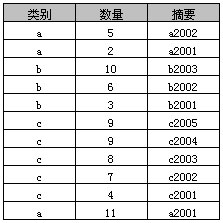### 简单Group By

```select 类别, sum(数量) as 数量之和
from A
group by 类别
```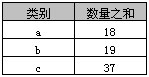### Group By 和 Order By

```select 类别, sum(数量) AS 数量之和
from A
group by 类别
order by sum(数量) desc
```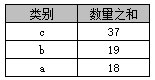### Group By中Select指定的字段限制

```select 类别, sum(数量) as 数量之和, 摘要
from A
group by 类别
order by 类别 desc
```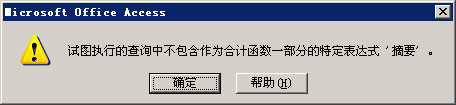### Group By All

```select 类别, 摘要, sum(数量) as 数量之和
from A
group by all 类别, 摘要
```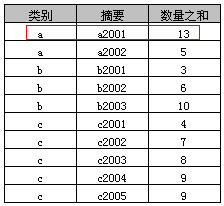“多列分组”实际上就是就是按照多列（类别+摘要）合并后的值进行分组，示例4中可以看到“a, a2001, 13”为“a, a2001, 11”和“a, a2001, 2”两条记录的合并。

SQL Server中虽然支持“group by all”，但Microsoft SQL Server 的未来版本中将删除 GROUP BY ALL，避免在新的开发工作中使用 GROUP BY ALL。Access中是不支持“Group By All”的，但Access中同样支持多列分组，上述SQL Server中的SQL在Access可以写成:

```select 类别, 摘要, sum(数量) AS 数量之和
from A
group by 类别, 摘要
```

### Group By与聚合函数

sum(列名) 求和
max(列名) 最大值
min(列名) 最小值
avg(列名)) 平均值
first(列名) 第一条记录 仅Access支持
last(列名) 最后一条记录 仅Access支持
count(列名) 统计记录数 注意和count(*)的区别

```select 类别, avg(数量) AS 平均值 from A group by 类别
```

```select 类别, count(*) AS 记录数 from A group by 类别
```

### Having与Where的区别

•where 子句的作用是在对查询结果进行分组前，将不符合where条件的行去掉，即在分组之前过滤数据，where条件中不能包含聚组函数，使用where条件过滤出特定的行。

having 子句的作用是筛选满足条件的组，即在分组之后过滤数据，条件中经常包含聚组函数，使用having条件过滤出特定的组，也可以使用多个分组标准进行分组。

```select 类别, sum(数量) as 数量之和 from A
group by 类别
having sum(数量) > 18
```

```select 类别, SUM(数量)from A
where 数量 gt;8
group by 类别
having SUM(数量) > 10
```

### Compute 和 Compute By

```select * from A where 数量 > 8
```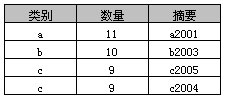```select *
from A
where 数量 > 8
compute max(数量),min(数量),avg(数量)
```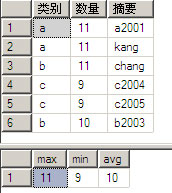compute子句能够观察“查询结果”的数据细节或统计各列数据（如例10中max、min和avg），返回结果由select列表和compute统计结果组成。

```select *
from A
where 数量 > 8
order by 类别
compute max(数量),min(数量),avg(数量) by 类别
```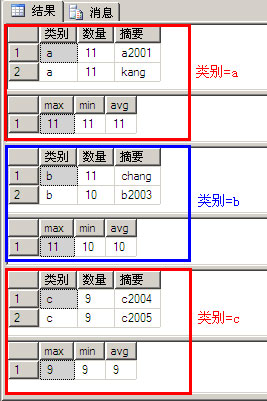• compute子句必须与order by子句用一起使用
• compute...by与group by相比，group by 只能得到各组数据的统计结果，而不能看到各组数据

• 暂无评论